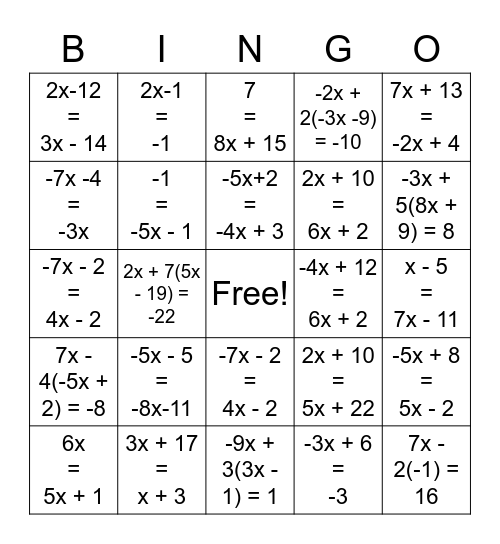# Substitution 1st step BingoThis bingo card has a free space and 24 words: -5x - 5 = -8x-11, 3x + 17 = x + 3, -5x+2 = -4x + 3, 6x = 5x + 1, 7x + 13 = -2x + 4, 2x-12 = 3x - 14, -7x -4 = -3x, 2x-1 = -1, -4x + 12 = 6x + 2, -7x - 2 = 4x - 2, 2x + 10 = 6x + 2, -7x - 2 = 4x - 2, 2x + 10 = 5x + 22, -5x + 8 = 5x - 2, -1 = -5x - 1, -3x + 6 = -3, x - 5 = 7x - 11, 7 = 8x + 15, -3x + 5(8x + 9) = 8, 2x + 7(5x - 19) = -22, 7x - 2(-1) = 16, 7x - 4(-5x + 2) = -8, -2x + 2(-3x -9) = -10 and -9x + 3(3x - 1) = 1.

## Play Online